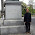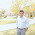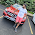VHDL coding tips and tricks: Positive edge triggered JK Flip Flop with reset input

## Sunday, March 28, 2010

### Positive edge triggered JK Flip Flop with reset input

Here is the code for JK Flip flop which is positive edge triggered.The flip flop also has a reset input which when set to '1' makes the output Q as '0' and Qbar as '1'.

--libraries to be used are specified here
library IEEE;
use IEEE.STD_LOGIC_1164.ALL;

--entity declaration with port definitions
entity JK_Flipflop is
port ( clk:     in std_logic;
J, K:               in std_logic;
Q, Qbar:       out std_logic;
reset:              in std_logic
);
end JK_Flipflop;

--architecture of entity
architecture Behavioral of JK_Flipflop is
--signal declaration.
signal qtemp,qbartemp : std_logic :='0';
begin
Q <= qtemp;
Qbar <= qbartemp;

process(clk,reset)
begin
if(reset = '1') then           --Reset the output.
qtemp <= '0';
qbartemp <= '1';
elsif( rising_edge(clk) ) then
if(J='0' and K='0') then       --No change in the output
NULL;
elsif(J='0' and K='1') then    --Set the output.
qtemp <= '0';
qbartemp <= '1';
elsif(J='1' and K='0') then    --Reset the output.
qtemp <= '1';
qbartemp <= '0';
else                           --Toggle the output.
qtemp <= not qtemp;
qbartemp <= not qbartemp;
end if;
end if;
end process;

end Behavioral;

The test bench program used for testing the design is given below:

--libraries to be used are specified here
library IEEE;
use IEEE.STD_LOGIC_1164.ALL;

entity testbench is
end testbench;

architecture behavior of testbench is
--Signal declarations
signal clk,J,K,reset,Q,Qbar : std_logic := '0';

-- Clock period definitions
constant clk_period : time := 1 ns;
begin
-- Instantiate the Unit Under Test (UUT)
UUT : entity work.JK_Flipflop port map (clk,J,K,Q,Qbar,reset);
-- Clock process definitions
clk_process :process
begin
clk <= '0';
wait for clk_period/2;
clk <= '1';
wait for clk_period/2;
end process;
-- Stimulus process
stim_proc: process
begin
J<='1';
K<='0';
wait for clk_period*2;

J<='1';
K<='1';
wait for clk_period*2;

J<='0';
K<='1';
wait for clk_period*2;

J<='0';
K<='0';
wait for clk_period*2;

J<='1';
K<='0';
wait for clk_period*2;

reset <='1';
J<='1';
K<='1';
wait for clk_period*2;

J<='0';
K<='1';
wait for clk_period*2;

reset <='0';
J<='1';
K<='1';
wait;
end process;

end;

The simulated waveform is shown below.Note that when reset is '1' the change in inputs doesn't affect the output.
The code was synthesized using XILINX ISE XST . The RTL schematic of the design is shown below:
In the schematic FDPE represents a single D-type flip-flop with data (D), clock enable (CE), and asynchronous preset (PRE) inputs and data output (Q). Similarly FDCE represents a single D-type flip-flop with data (D), clock enable (CE), and asynchronous clear (CLR) inputs and data output (Q).

Note :- Use RTL Viewer to get a closer look on how your design is implemented in hardware.

#### 8 comments:

1.Q <= qtemp;
Qbar <= qbartemp; you placed it above the process statement, is any thing wrong if i placed it after end if statements?
can u explain the simulation of the above architecture body.(what i want to know is like compilation steps in 'C')

2.@ashok : If you put them inside the process statement then, it gets executed only on clock edges. Also you may get a synthesis error.

It is better to place those statements outside the process.Because we are assigning the temporary variable values to output signals, and we want to update the values immediately.

3.Why doesn't XST infer a single DFF with preset and clear?

4.Why did you use qtemp and qbartemp? Can I use Q and Qbar directly in the process? Thanks ^^

1.I asked the same question

2.In vhdl, you cannot operate on the outputs. For example , this statement wouldnt be allowed:
q <= not q;
qbar <= not qbar;

5.i think Q and Qbar should be inout_std_logic in entity.......

6.we need conclusion for the above code